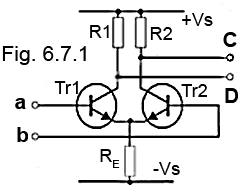# Op Amps Quiz

Try our quiz, based on the information you can find in Amplifiers Module 6 Submit your answers and see how many you get right. If you get any answers wrong. Just follow the hints to find the right answer and learn about Amplifiers as you go.

### 1.

Which of the following properties should an ideal op amp have?

### 2.In Fig. 6.7.1, Tr2 is conducting due to a fixed voltage at input ‘b’. If input ‘a’ becomes more positive than ‘b’, what will be the resulting change at outputs C & D?

### 3.

On op amps that use an offset null control, this is adjusted to give which of the following conditions:

### 4.

Calculate the approximate voltage gain of the amplifier in Fig 6.7.2### 5.

Refer to Fig 6.7.2 : If R2 is changed to 82K and +0.7 V DC (w.r.t. 0V) is applied to the circuit input, what will be the approximate output voltage and its polarity w.r.t 0V?

### 6.

What does the "Large Signal Voltage Gain" of an op amp refer to?

### 7.

What characteristic of an op amp is the formula 2πƒ Vpk used to calculate?

### 8.

Refer to Fig 6.7.3, which shows the DC input to pin 3, the low frequency signal to pin 2 and the output signal at pin 6 of a 741 op amp. What purpose is the op amp being used for?### 9.

Fig. 6.7.4 shows the package outline of a TLC271 programmable op amp. If the two ends of a preset potentiometer are connected across pins 1 and 5, to which pin should the potentiometer wiper be connected to?### 10.

Simple D to A conversion can be achieved using a weighted series of resistors and a single op amp configured in which of the following modes?

Top of Page